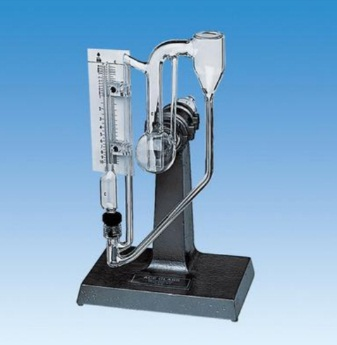# What is McCLEOD GAUGE## Introduction

McCLEOD GAUGE is generally used as a primary standard for the measurement of high vacuum (low pressure) and is simple to use. It was invented in 1874 by Herbert McCleod.

## Principle

McLeod gauges operate by taking in a sample volume of gas from a vacuum chamber, and then compressing it by tilting, and infilling with mercury. The pressure in this smaller volume is then measured by a mercury manometer, and, by knowing the compression ratio, the pressure of the original vacuum can be determined.

The gas used must obey Boyle’s law given by; P1V1=P2V2
where, P1 = Pressure of gas at initial condition (applied pressure).
P2 = Pressure of gas at final condition.
V1 = Volume of gas at initial Condition.
V2 = Volume of gas at final Condition.

## ConstructionThe main parts of McLeod gauge are as follows:
A reference column with reference capillary tube. The reference capillary tube has a point called
zero reference point. This reference column is connected to a bulb and measuring capillary and the place of connection of the bulb with reference column is called as cut off point. (It is called
the cut off point, since if the mercury level is raised above this point, it will cut off the entry of
the applied pressure to the bulb and measuring capillary. Below the reference column and the
bulb, there is a mercury reservoir operated by a piston.

## Working

The McLeod gauge is operated as follows:
The pressure to be measured (P1) is applied to the top of the reference column of the McLeod Gauge as shown in diagram. The mercury level in the gauge is raised by operating the piston to fill the volume as shown by the dark shade in the diagram. When this is the case (condition – 1), the applied pressure fills the bulb and the capillary. Now again the piston is operated so that the mercury level in the gauge increases. When the mercury level reaches the cutoff point, a known volume of gas (V1) is trapped in the bulb and measuring capillary tube. The mercury level is further raised by operating the piston so the trapped gas in the bulb and measuring capillary tube are compressed. This is done until the mercury level reaches the “Zero reference Point” marked on the reference capillary (condition – 2). In this condition, the volume of the gas in the measuring capillary tube is read directly by a scale besides it. That is, the difference in height ‘H’ of the measuring capillary and the reference capillary becomes a measure of the volume (V2) and pressure (P2) of the trapped gas.

Now as V1, V2 and P2 are known, the applied pressure P1 can be calculated using Boyle’s Law given by; P1V1 = P2V2

Let the volume of the bulb from the cutoff point upto the beginning of the measuring capillary  tube = V
Let area of cross – section of the measuring capillary tube = a
Let height of measuring capillary tube = hc.
Therefore, Initial Volume of gas entrapped in the bulb plus measuring capillary tube = V1 = V+ahc.
When the mercury has been forced upwards to reach the zero reference point in the reference capillary, the final volume of the gas = V2 +ah.
Where, h = height of the compressed gas in the measuring capillary tube
P1 = Applied pressure of the gas unknown.
P2 = Pressure of gas at final condition, that is, after compression = P1+h

### Applications

The McLeod Gauge is used to measure vacuum pressure.

### Advantages of the McLeod Gauge

(i) It is independent of the gas composition.
(ii) It serves as a reference standard to calibrate other low pressure gauges.
(iii) A linear relationship exists between the applied pressure and h
(iv) There is no need to apply corrections to the McLeod Gauge readings.

### Limitations of McLeod Gauge

(i) The gas whose pressure is to be measured should obey the Boyle’s law
(ii) Moisture traps must be provided to avoid any considerable vapor into the gauge.
(iii) It measure only on a sampling basis.
(iv) It cannot give a continuous output